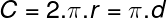# Circle area calculator, formulas, practical use.

## Circle area and circumference calculations

The circle area calculator is designed for calculations of radius, diameter, circumference or area of a circle. For successful calculation, you need to know and enter into the calculator at least one of parameters. Then press send or enter to run calculations.

## Parameters sketch:﻿
Unit system:

## Variables and constants explanation:

C – circumference
d – diameter
A – area
π– 3,1415926535
– unspecified values
specified values
calculated values

## Circle circumference calculation formula:## Circle area calculation formula:## Circle area calculator use:

Circle calculation is used in many industries and in a huge number of calculations. This is at least some of them:

• perimeter of a tree trunk, limb, twig, stem, pipe, tube, hose, duct, wire, rod, cord, thread, rope, tunnel, pillar
• cross-sectional area of a tree trunk, limb, twig, stem, pipe, tube, hose, duct, wire, rod, cord, thread, rope, tunnel, pillar
• partial calculations of geometric shapes like cilinder, cone, sphere, barrel, torus and other rotational shapes
• school calculations